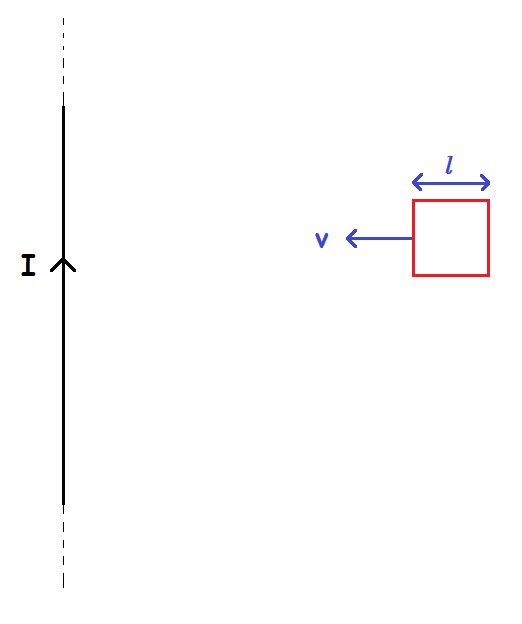# A square frame projected towards an infinite current carrying wire.A square frame of length $l=1$ m, mass $m= 1$ g, resistance $R=1 \Omega$ is projected from a large distance towards a fixed infinitely long wire carrying current $I= 1$ kAmp with a speed $v_{0} = 10$ m/s.

Let $a$ be the total distance of the left part of frame from wire in metres when the frame comes to rest.

Find $\displaystyle \int_{a}^{\infty} \dfrac{dx}{(x(1+x))^2}$

×